# Inventory management – Stochastic supply

This entry is part 4 of 4 in the series Predictive Analytics

### Introduction

We will now return to the newsvendor who was facing a onetime purchasing decision; where to set the inventory level to maximize expected profit – given his knowledge of the demand distribution.  It turned out that even if we did not know the closed form1 of the demand distribution, we could find the inventory level that maximized profit and how this affected the vendor’s risk – assuming that his supply with certainty could be fixed to that level. But what if that is not the case? What if the supply his supply is uncertain? Can we still optimize his inventory level?

We will look at to slightly different cases:

1.  one where supply is uniformly distributed, with actual delivery from 80% to 100% of his ordered volume and
2. the other where the supply have a triangular distribution, with actual delivery from 80% to 105% of his ordered volume, but with most likely delivery at 100%.

The demand distribution is as shown below (as before):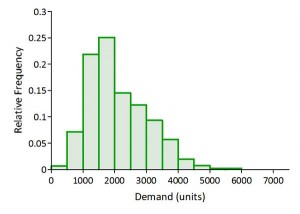### Maximizing profit – uniformly distributed supply

The figure below indicates what happens as we change the inventory level – given fixed supply (blue line). We can see as we successively move to higher inventory levels (from left to right on the x-axis) that expected profit will increase to a point of maximum.

If we let the actual delivery follow the uniform distribution described above, and successively changes the order point expected profit will follow the red line in the graph below. We can see that the new order point is to the right and further out on the inventory axis (order point). The vendor is forced to order more newspapers to ‘outweigh’ the supply uncertainty: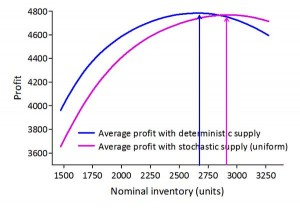At the point of maximum profit the actual deliveries spans from 2300 to 2900 units with a mean close to the inventory level giving maximum profit for the fixed supply case: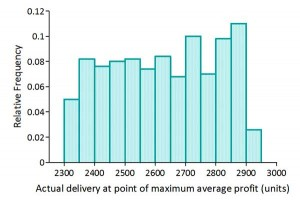The realized profits are as shown in the frequency graph below: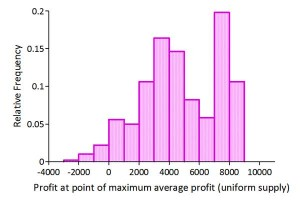Average profit has to some extent been reduced compared with the non-stochastic supply case, but more important is the increase in profit variability. Measured by the quartile variation, this variability has increased by almost 13%, and this is mainly caused by an increased negative skewness – the down side has been raised.

### Maximizing profit – triangular distributed supply

Again we compare the expected profit with delivery following the triangular distribution as described above (red line) with the expected profit created by known and fixed supply (blue line).  We can see as we successively move to higher inventory levels (from left to right on the x-axis) that expected profits will increase to a point of maximum. However the order point for the stochastic supply is to the right and further out on the inventory axis than for the non-stochastic case: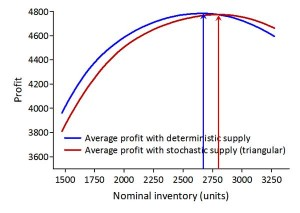The uncertain supply again forces the vendor to order more newspapers to ‘outweigh’ the supply uncertainty: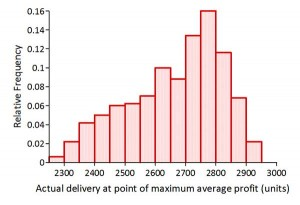At the point of maximum profit the actual deliveries spans from 2250 to 2900 units with a mean again close to the inventory level giving maximum profit for the fixed supply case2 .

The realized profits are as shown in the frequency graph below: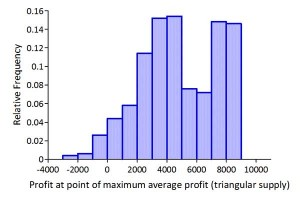Average profit has somewhat been reduced compared with the non-stochastic supply case, but more important is the increase in profit variability. Measured by the quartile variation this variability has increased by 10%, and this is again mainly caused by an increased negative skewness – again have the down side been raised.

The introduction of uncertain supply has shown that profit can still be maximized however the profit will be reduced by increased costs both in lost sales and in excess inventory. But most important, profit variability will increase raising issues of possible other strategies.

### Summary

We have shown through Monte-Carlo simulations, that the ‘order point’ when the actual delivered amount is uncertain can be calculated without knowing the closed form of the demand distribution. We actually do not need the closed form for the distribution describing delivery, only historic data for the supplier’s performance (reliability).

Since we do not need the closed form of the demand distribution or supply, we are not limited to such distributions, but can use historic data to describe the uncertainty as frequency distributions. Expanding the scope of analysis to include supply disruptions, localization of inventory etc. is thus a natural extension of this method.

This opens for use of robust and efficient methods and techniques for solving problems in inventory management unrestricted by the form of the demand distribution and best of all, the results given as graphs will be more easily communicated to all parties than pure mathematical descriptions of the solutions.

Average profit has to some extent been reduced compared with the non-stochastic supply case, but more important is the increase in profit variability. Measured by the quartile variation, this variability has increased by almost 13%, and this is mainly caused by an increased negative skewness – the down side has been raised.

Series Navigation<< Inventory management – Some effects of risk pooling
1. In mathematics, an expression is said to be a closed-form expression if it can be expressed analytically in terms of a finite number of certain “well-known” functions. []
2. This is not necessarily true for other combinations of demand and supply distributions. []